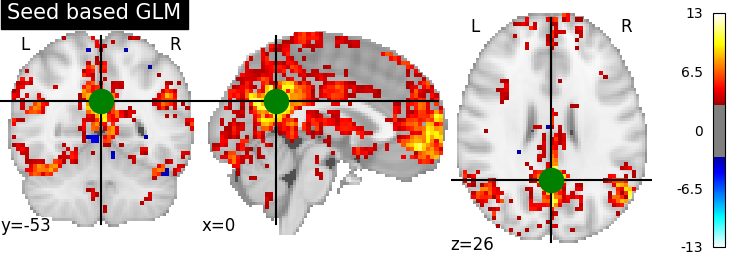# 2.2.2. Default Mode Network extraction of AHDH dataset¶

This example shows a full step-by-step workflow of fitting a GLM to data extracted from a seed on the Posterior Cingulate Cortex and saving the results.

More specifically:

1. A sequence of fMRI volumes are loaded.

2. A design matrix with the Posterior Cingulate Cortex seed is defined.

3. A GLM is applied to the dataset (effect/covariance, then contrast estimation).

4. The Default Mode Network is displayed.

```import numpy as np

from nilearn import datasets, plotting

from nistats.first_level_model import FirstLevelModel
from nistats.design_matrix import make_first_level_design_matrix
```

## 2.2.2.1. Prepare data and analysis parameters¶

Prepare the data.

```adhd_dataset = datasets.fetch_adhd(n_subjects=1)

# Prepare timing
t_r = 2.
slice_time_ref = 0.
n_scans = 176

# Prepare seed
pcc_coords = (0, -53, 26)
```

Out:

```/home/kshitij/miniconda3/envs/nistats-py36-latest/lib/python3.6/site-packages/nilearn/datasets/func.py:516: VisibleDeprecationWarning: Reading unicode strings without specifying the encoding argument is deprecated. Set the encoding, use None for the system default.
dtype=None)
```

## 2.2.2.2. Estimate contrasts¶

Specify the contrasts.

```seed_masker = NiftiSpheresMasker([pcc_coords], radius=10, detrend=True,
standardize=True, low_pass=0.1,
high_pass=0.01, t_r=2.,
memory='nilearn_cache',
memory_level=1, verbose=0)
frametimes = np.linspace(0, (n_scans - 1) * t_r, n_scans)
design_matrix = make_first_level_design_matrix(frametimes, hrf_model='spm',
dmn_contrast = np.array( + *(design_matrix.shape-1))
contrasts = {'seed_based_glm': dmn_contrast}
```

## 2.2.2.3. Perform first level analysis¶

Setup and fit GLM.

```first_level_model = FirstLevelModel(t_r=t_r, slice_time_ref=slice_time_ref)
design_matrices=design_matrix)
```

Out:

```/home/kshitij/workspace/nistats-org/nistats-repo/nistats-kchawla-pi/nistats/first_level_model.py:73: UserWarning: Mean values of 0 observed.The data have probably been centered.Scaling might not work as expected
warn('Mean values of 0 observed.'
```

Estimate the contrast.

```print('Contrast seed_based_glm computed.')
z_map = first_level_model.compute_contrast(contrasts['seed_based_glm'],
output_type='z_score')

# Saving snapshots of the contrasts
filename = 'dmn_z_map.png'
display = plotting.plot_stat_map(z_map, threshold=3.0, title='Seed based GLM',
cut_coords=pcc_coords)
display.savefig(filename)
print("Save z-map in '{0}'.".format(filename))
```Out:

```Contrast seed_based_glm computed.
Save z-map in 'dmn_z_map.png'.
```

## 2.2.2.4. Generating a report¶

It can be useful to quickly generate a portable, ready-to-view report with most of the pertinent information. This is easy to do if you have a fitted model and the list of contrasts, which we do here.

```from nistats.reporting import make_glm_report

report = make_glm_report(first_level_model,
contrasts=contrasts,
cluster_threshold=15,
min_distance=8.,
plot_type='glass',
)
```

We have several ways to access the report:

```# report  # This report can be viewed in a notebook
# report.save_as_html('report.html')
# report.open_in_browser()
```

Total running time of the script: ( 0 minutes 8.361 seconds)

Gallery generated by Sphinx-Gallery